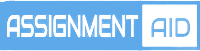# Applied Statistics Homework Help#### What Is Applied Statistics

Statistics is broadly classified into two groups namely; Theoretical and Applied Statistics. Theoretical statistics also called mathematical statistics mainly involves the derivation of statistical theorems, the development of standard formulas, rules, and laws to be used for solving many real-world problems. Applied statistics on the other hand mainly deals with the applications of theorems and related concepts developed in theoretical statistics in the area of Business, Research and Development Units, Government Sectors, Marketing Research, Clinical Trials, etc. It is the collection, analysis using statistical algorithms and in some instances software and machine learning techniques, interpretation, organization, and presentation of data in a visual way that is easy to understand.

Applied statistics is divided into two categories namely;

1.  Descriptive Statistics

This branch of applied statistics mainly focuses on the quantitative description of statistical data. The major significance of descriptive statistics is that it largely summarizes massive data and gives easy visualization of the data making it very easy to understand and come up with quick decisions. In descriptive statistics, data can be summarized in two ways. One way is the use of summary graphs and tables/charts. These give a quantitative overview of the data and make it simple to draw quick conclusions by just taking a glance. The other way is to use univariate analysis techniques or numerical. These include measures of central tendency and measures of dispersion like mean, variance, average, mode, meridian, etc.

1. Inferential Statistics.

Inferential statistics focuses on the use of data analysis techniques to find out the underlying characteristics of the population. Unlike descriptive statistics which is focused on the observable characteristic, inferential statistics are more of estimates. This branch of statistics deals with making conclusions about the whole population based on the corresponding sample information. The goal is to make inferences about the population characteristics based on the analysis of sample data drawn from this population.

From the two branches, the following are some of the topics in applied statistics

1. Probability and Probability Distributions
2. Hypothesis testing
3. Analysis of Variance
4. Multinomial experiments and Contingency tables
5. Univariate variables
6. Estimates and samples
7. Correlation and regression

Softwares used to perform statistical analysis

#### Some Of The Software Used To Perform Analysis In Applied Statistics

1. STATA. This is a statistical software package that is used to analyze data, manage data, and create graphics. This is used by many researchers who are carrying out research in different fields to examine the data patterns thoroughly.
2. SPSS. This is a software package that is used to analyze and compute statistical information.
3. SAS. This is the programming language that is used to store data from any of the resources that would have the format similar to your database
4. PHStat. This is the widely used software that uses Microsoft Excel to explore statistics. This is used for regression analysis, summarization of data, and un-summarization of data.
5. Megastat: This is an add-on to the Excel that will carry out statistical analysis using an Excel workbook. This carries out basic operations like descriptive statistics, frequency distribution, probability calculation, and hypothesis testing.

#### Applied Statistics Homework Help

Studying applied statistics involves many formulas and algorithms that in most cases are closely related. In most cases, therefore, most students end up confused about which formula to apply where when they encounter a question in the subject. Applied statistics also involves the use of software packages mostly in colleges with a majority of students being not well equipped to use these software to solve problems.

Therefore handling applied statistics assignment calls for a good mastery of the formulas as well as being good on the use of the software. For many students, real trouble kicks in at the data analysis stage where they are always required to implement algorithms that require massive amounts of knowledge and advanced computer skills. However, this should not scare you as our expert writers are just a click away. They are well skilled and equipped to handle your applied statistics homework at a reasonable rate. Reach us today for quality applied statistics homework help services.

## Example Samples

live:topwrit

###### Email:

support@assignmentsaid.com

###### Open Hours:

Mon-Sat: 9am - 6pm